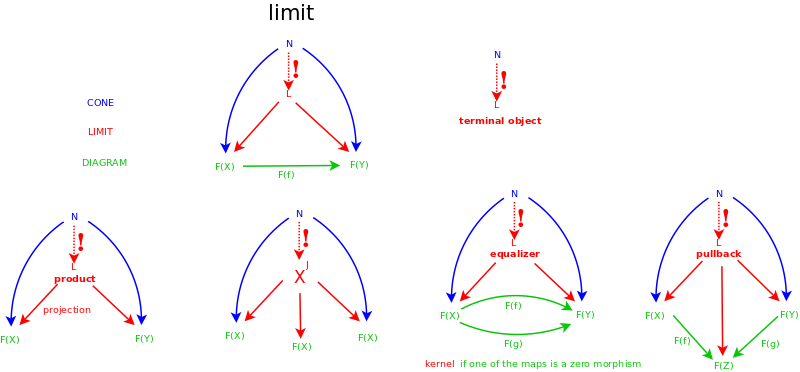• ms@ms.lt
• +370 607 27 665
• My work is in the Public Domain for all to share freely.

Introduction E9F5FC

Understandable FFFFFF

Questions FFFFC0

Notes EEEEEE

Software

Study:

Natural transformations

• If a functor takes us from a syntactic category to a semantic category, then what does the adjoint functor mean?
• How is "extending the domain" related to adjunction?
• How do the six operations match the six criteria?
• How to deal with self-identity or non-identity f of an M-category? with copies of an M-category? Perhaps by embedding it in a bigger system?

Equality

• At what level is equality defined in defining a category? Equality is needed for the properties of identity and associativity. But is it the same identity as the identity for other morphisms?

I'm trying to learn category theory because it is relevant for homotopy type theory and other areas of mathematics. But also I may be able to use it to model parts of my philosophy.

Category theory concepts such as adjoints (least upper bounds, greatest lower bounds) and limits-colimits are actually concepts of analysis.

Category theory externalizes the internal structure of mathematical objects. It reexpresses that internal structures in terms of constraints on external relationships between structures.

Category theory relates God's outer perspective (on the general, external "black box" relationships) and our inner perspective, within the system, in terms of the properties of our particular system. The question of God's necessity and nature includes the relationship between God and human's perspectives.

Category theory expresses a duality between objects and mappings. Thus functors likewise have this dual nature, and so do natural transformations.

Category Theory Videos

Category Theory Books and ArticlesIdeas

• Categorification (making math explicit) vs. Decategorification (making math implicit). Algebraic combinatorics is the concrete flip-side of the abstractness of category theory. But algebraic combinatorics comes with implicit interpretation whereas category theory comes with explicit notation.
• Categorical models for psychological consciousness. Sheaf theory - consciousness.
• In the mathematical ways of figuring things out: Multiset is What, Set is How, List is Why. The reason that Set Theory works is that it is based on How, which is the level for all answers.

For each object {$x$}, the identity morphism {${id}_x$} must be unique. Because consider {${id1}_x \circ {id2}_x$}. The identity is whichever disappears.

I am thinking that categories should be considered on three levels:

• Objects (of being - what is)
• Arrows (of doing)
• Equations (of reflecting) that relate arrows (or objects), especially in composition.

The composition of the arrows always seems to me underexplained. And that is where the different levels of equivalence become relevant. Also to be considered is whether an object should be thought of as an arrow to itself.

Functors

• A functor is defined by what it does on a composition triangle of morphisms, and what it does on the identity morphism: {$F(a \overset{f}{\rightarrow} b \overset{g}{\rightarrow} c) \Rightarrow F(a) \overset{F(f)}{\rightarrow} F(b) \overset{F(g)}{\rightarrow} F(c)$}
• A functor is an interpretation that takes us from a syntax category to a semantics category.
• Milewski: A functor embeds one category in another.
• Milewski: A functor may collapse multiple objects/functions into one, but it never breaks connections.

Natural transformations

• Given functors F and G, both from C to D, a natural transformation {$eta$} maps every particular object X in C to a particular morphism {$eta_X$} from {$F(x)$} to {$G(x)$}. In this sense, the object is why (as a generalization of how) and the morphism is whether (as a generalization of what). Why and whether hold beyond circumstances (the functor), whereas how and what make sense within circumstances (the functor).
• Natural transformations don't depend on the structure internal to the objects, but only on their external relationships, as expressed by the category.
• The components of natural transformations depend only on the objects. If you know these components, then the morphisms carry over automatically.
• Natural transformations say that the trivially existing bijection (between FA and GA, FB and GB) is actually a morphism in the category D.

Examples

• Z ir R. Include Z in R. Get floor of element in R to go back.
• Return and extract in Haskell.
• Expanding (replacing Identities with L*R) and collapsing (replacing L*R with Identity).
• See Wikipedia: Currying {$B \mapsto B\times C$} is left adjoint to {$A \mapsto A^C$}. This grounds the equation {$A^{B\times C}\cong (A^C)^B$}.

Relate id-A to consciousness, to constancy of attention, recurring attention.

Significant=unencompassable.

Covering=encompassing=Why.

Computation trinitarianism takes as equivalent a proof of a proposition, a program with output of some type, and a generalized element of some object.

Notes

• Kategorijų teorijoje: nagrinėti įvairių lygmenų klaidas.
• Adjunction is like extension of the domain, but in terms of structure: extension of structure. Polymorphism. Try to relate Z and Q and R and other examples.

CategoryTheory

Naujausi pakeitimai

 Puslapis paskutinį kartą pakeistas 2019 balandžio 13 d., 17:56